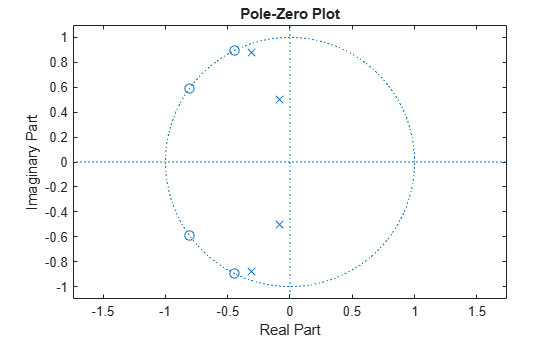zplane

Z-plane zero-pole plot for discrete-time filter System object

Description

example

zplane(sysobj) plots the zeros and poles of the filter System object™, sysobj, with the unit circle for reference in the Filter Visualization Tool (FVTool). Each zero is represented with an 'o' and each pole with a 'x' on the plot. Multiple zeros and poles are indicated by the multiplicity number shown at the upper right of the zero or pole.

When you run the filter System object with a fixed-point input, the filter becomes a quantized fixed-point filter, filtQuant. When filtQuant is a quantized filter, zplane(filtQuant) plots the poles and zeros of the quantized and unquantized filters. The symbolsand + represent the zeros and poles of the quantized filter filtQuant. The plot includes the unit circle for reference.

zplane(sysobj,'Arithmetic',arithType) also analyzes the filter System object, sysobj, based on the arithmetic specified in arithType.

[z,p,k] = zplane(sysobj) returns the zeros, poles, and the gain of the filter.

For more input options, see zplane in Signal Processing Toolbox™.

Examples

collapse all

Create a fourth-order IIR digital filter with a cutoff frequency of 0.6. Plot the poles and zeros of this filter.

[b,a] = ellip(4,.5,20,.6);
zplane(b,a)Quantize the filter by passing a fixed-point input through the filter algorithm. Plot the quantized and unquantized poles and zeros associated with this filter.

iirFilt = dsp.IIRFilter('Numerator',b,'Denominator',a);
in = fi(randn(15,6),1,15,3);
out = iirFilt(in);
zplane(iirFilt)Input Arguments

collapse all

Arithmetic used in the filter analysis, specified as 'double', 'single', or 'Fixed'. When the arithmetic input is not specified and the filter System object is unlocked, the analysis tool assumes a double-precision filter. When the arithmetic input is not specified and the System object is locked, the function performs the analysis based on the data type of the locked input.

The 'Fixed' value applies to filter System objects with fixed-point properties only.

When the 'Arithmetic' input argument is specified as 'Fixed' and the filter object has the data type of the coefficients set to 'Same word length as input', the arithmetic analysis depends on whether the System object is unlocked or locked.

• unlocked –– The analysis object function cannot determine the coefficients data type. The function assumes that the coefficients data type is signed, has a 16-bit word length, and is auto scaled. The function performs fixed-point analysis based on this assumption.

• locked –– When the input data type is 'double' or 'single', the analysis object function cannot determine the coefficients data type. The function assumes that the data type of the coefficients is signed, has a 16-bit word length, and is auto scaled. The function performs fixed-point analysis based on this assumption.

To check if the System object is locked or unlocked, use the isLocked function.

When the arithmetic input is specified as 'Fixed' and the filter object has the data type of the coefficients set to a custom numeric type, the object function performs fixed-point analysis based on the custom numeric data type.

Output Arguments

collapse all

Location of zeros of the filter transfer function on the zplane, returned as a column vector. The zeros are the roots of the polynomial whose coefficients are given by the numerator coefficients of the filter System object.

In case there are no zeros, the vector z is set to an empty matrix [].

Data Types: double
Complex Number Support: Yes

Location of poles of the filter transfer function on the zplane, returned as a column vector. The poles are the roots of the polynomial whose coefficients are given by the denominator coefficients of the filter System object.

In case there are no poles, the vector p is set to an empty matrix [].

Data Types: double
Complex Number Support: Yes

Gain of the filter, returned as a real scalar.

Data Types: double